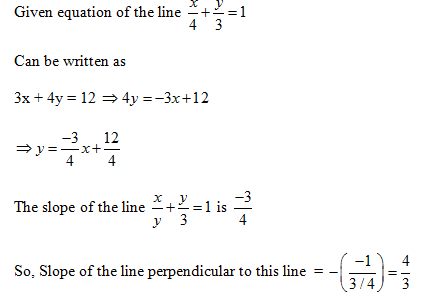# SSC CGL EXAMS 2018 | Quantitative Aptitude Practice Questions (Day-6)

Dear Aspirants, Here we have given the Important SSC Exam 2018 Practice Test Papers. Candidates those who are preparing for SSC 2018 can practice these questions to get more confidence to Crack SSC 2018 Examination.

[WpProQuiz 4125]

1) The average of a batsman for 40 innings is 50 runs. His highest score exceeds his lowest score by 172 runs. If these two innings are excluded, his average drops by 2 runs. Find his higher score.

a) 172

b) 173

c) 174

d) 175

2) A butler stores wine from a butt of sherry which contained 30% of spirit and he replaced what he had stolen by wine containing only 12% of spirit. The butt was then 18% strong only. How much of the butt did he steal?

a) 1/3

b) 2/5

c) 2/3

d) 4/7

3) Madan pays income tax at the rate of 10%. If his income increased by 10% and his tax rate increases to 15%. His net income would increase by Rs.350. What is Madan’s income?

a) 8,000

b) 10,000

c) 12,000

d) 14,000

4) A shopkeeper purchases a packet of 50 pens at Rs.10 per pen. He sells a part of the packet at a profit of 30%. On the remaining part, he incurs a loss of 10%. If his overall profit on the whole packet is 10%, the number of pens he sold at profit is:

a) 25

b) 30

c) 20

d) 15

5) Two equal sums of money were invested, one at 4% and the other at 4.5%. At the end of 7 years, the simple interest received from the latter exceeded to that received from the former by Rs 31.50. Each sum was

a) Rs 1200

b) Rs 600

c) Rs 750

d) Rs 900

6) If a : b = c : d and e : f = g : h, then (ae = bf) : (ae – bf) = ?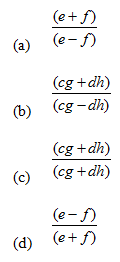7) 4 pipes each of 3 cm diameter are to be replaced by a single pipe discharging the same quantity of water. What should be the diameter of the single pipe, if the speed of water is the same?

a) 2 cm

b) 4 cm

c) 6 cm

d) 8 cm

8) A man sitting in a train travelling at the rate of 50 km/hr observes that it takes 9 sec for a goods train travelling in the opposite direction to pass him. If the goods train is 187.5 m long, find its speed.

a) 40 km/hr

b) 25 km/hr

c) 35 km/hr

d) 36 km/hr

9) If a + b = 1, then a4 + b4 – a³ – b³ – 2a²b² + ab is equal to

a) 1

b) 2

c) 0

d) 4

10) If is a perfect square where a and b are positive real numbers, then the value of a and b

a) a = 5, b = 6

b) a = 6, b = 7

c) a = 7, b = 7

d) a = 7, b = 8

11)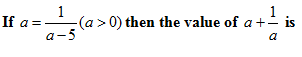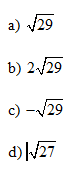12) If cos θ = (x2 – y2)/(x2 + y2), then the value of cotθ is [if 0≤θ≤90° ]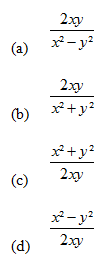13) If x = cosecq – sinq and y = secq – cosq, then the relation between x and y is

a) x² + y² + 3 = 1

b) x²y²(x²+y²+3) = 1

c) x²(x² + y² – 5) = 1

d) y²(x² + y² – 5) = 1

14) The set of values of p for which the roots of the equation 3x² + 2x + p(p – 1) = 0 are the opposite sign is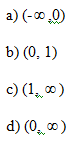15) What is the slope of the line perpendicular to the line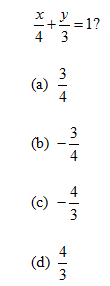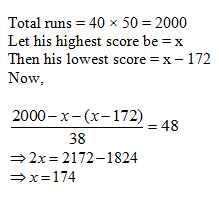By the alligation rule, we find that wine containing 30% of spirit and wine containing 12% of spirit should be mixed in the ratio 1: 2 to produce a mixture containing 18% of spirit.

Ratio = 6:12 = 1:2

This means that 1/3rd of the butt of sherry was left, i.e. to say, the butler drew out 2/3rd of the butt. 2/3rd of the butt was stolen.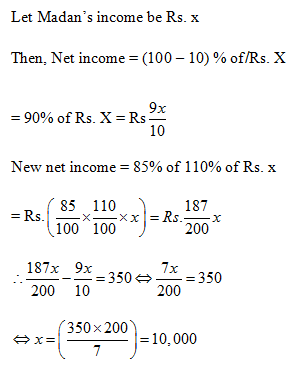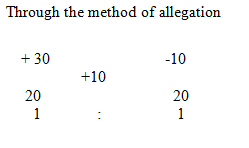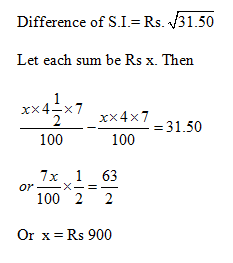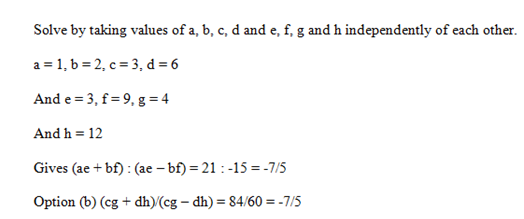Let h be the length of water column discharged in 1 hour or 1 minute

Volume discharged by the 4 pipes

= Volume discharged by the single pipe.

= 4 × p × (1.5)² × h = p × (r)² × h

r² = 9

r = 3, Diameter = 6 cm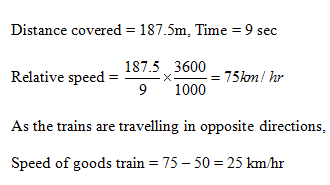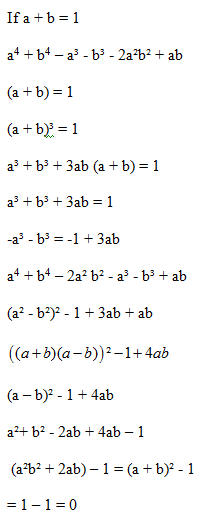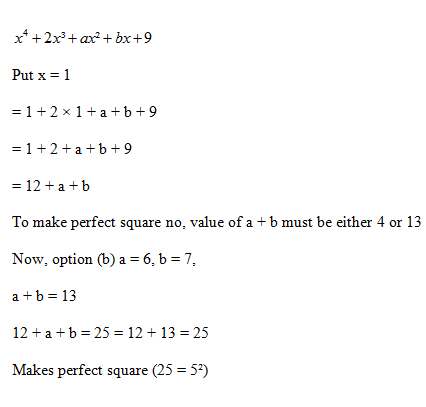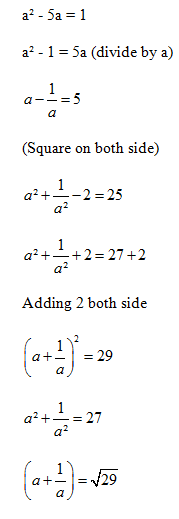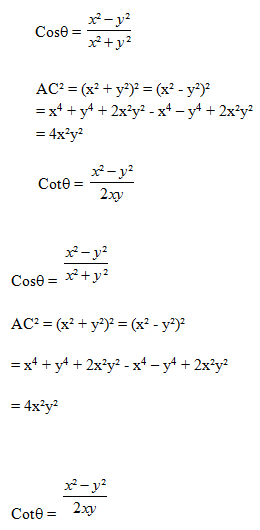x = cosecq – sinq

y=secq – cosq

Put q = 45º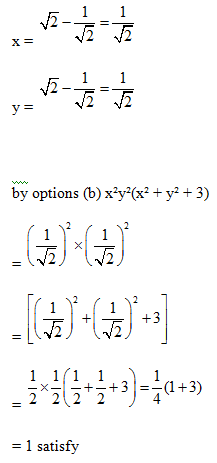p (p – 1)/3 < 0 (product of roots should be negative)

p(p – 1) < 0

p² – p < 0

This happens for 0 < p < 1